Question

# A car of mass M = 1300 kg traveling at 65.0 km/hour enters a banked turn...

A car of mass M = 1300 kg traveling at 65.0 km/hour enters a banked turn covered with ice. The road is banked at an angle θ, and there is no friction between the road and the car's tires as shown in (Figure 1) . Use g = 9.80 m/s2 throughout this problem. r= 91.43 m. Now, suppose that the curve is level (θ=0) and that the ice has melted, so that there is a coefficient of static friction μ between the road and the car's tires as shown in (Figure 2) . What is μmin, the minimum value of the coefficient of static friction between the tires and the road required to prevent the car from slipping? Assume that the car's speed is still 65.0 km/hour and that the radius of the curve is 91.4 m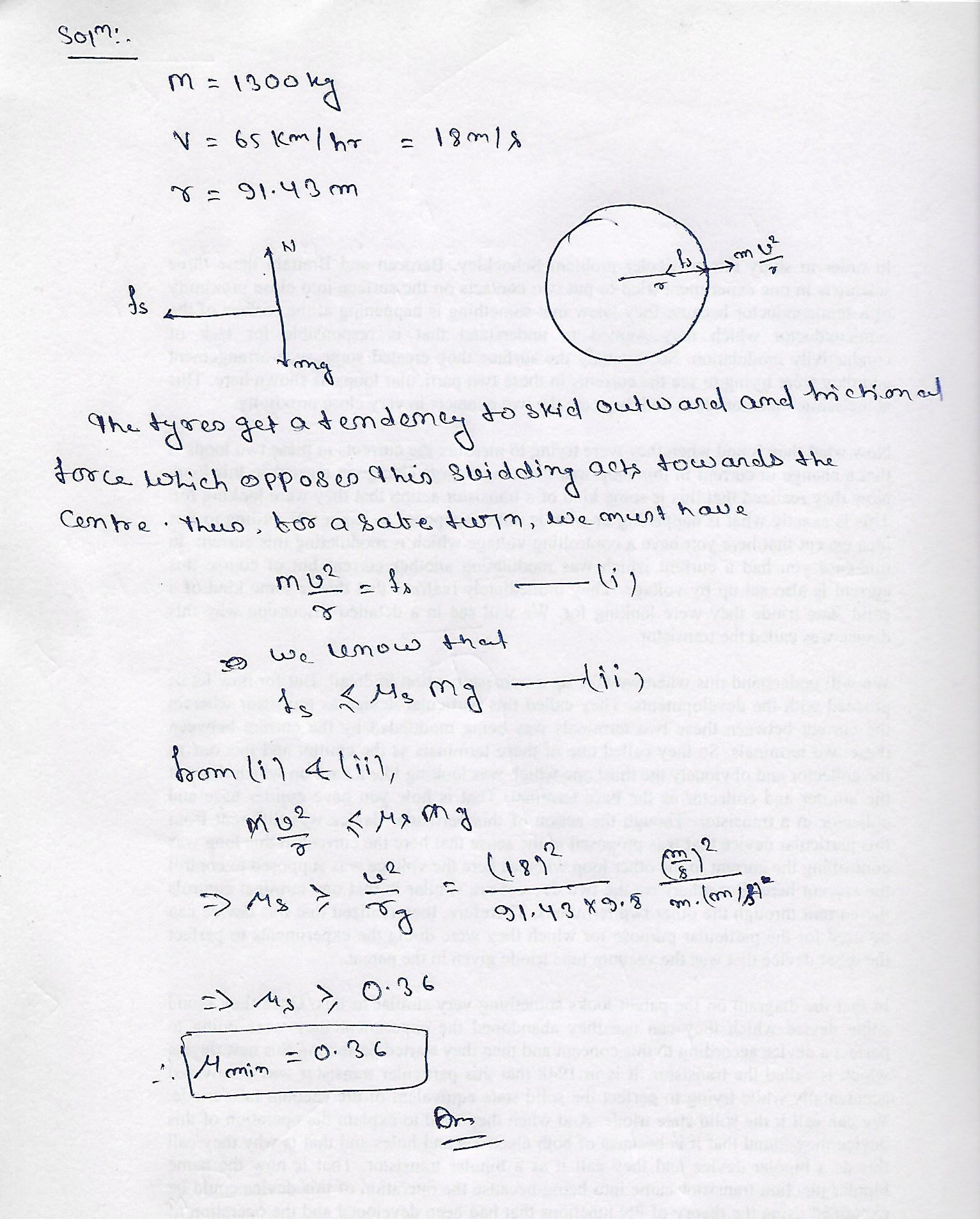#### Earn Coins

Coins can be redeemed for fabulous gifts.

Similar Homework Help Questions
• ### A car of mass M = 800 kg traveling at 55.0 km/hour enters a banked turn...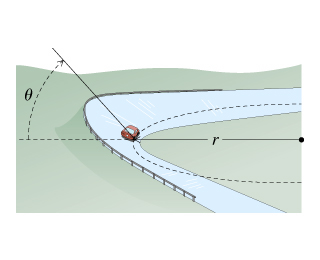A car of mass M = 800 kg traveling at 55.0 km/hour enters a banked turn covered with ice. The road is banked at an angle ?, and there is no friction between the road and the car's tires as shown in(Figure 1) . Use g = 9.80 m/s2 throughout this problem. Now, suppose that the curve is level (?=0) and that the ice has melted, so that there is a coefficient of static friction ? between the road and...

• ### A car of mass M = 1500 kg traveling at 65.0 km/hour enters a banked turn...

A car of mass M = 1500 kg traveling at 65.0 km/hour enters a banked turn covered with ice. The road is banked at an angle ∘, and there is no friction between the road and the car's tires as shown in (Figure 1). Use g = 9.80 m/s2 throughout this problem. What is the radius r of the turn if θ = 20.0 ∘ (assuming the car continues in uniform circular motion around the turn)?

• ### A car of mass M= 1300 kg traveling at 65.0 km/hour enters a banked turn covered with ice. The road is banked at an ang...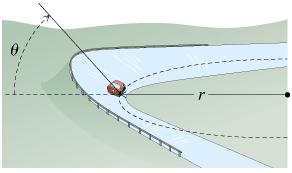A car of mass M= 1300 kg traveling at 65.0 km/hour enters a banked turn covered with ice. The road is banked at an angle , and there is no friction between the road and the car's tires. (Intro 1 figure) . Use g= 9.80 m/s^2 throughout this problem. What is the radius (in meters) of the turn if = 20.0 (assuming the car continues in uniform circular motion around the turn)?

• ### A car of mass M = 1300 kg traveling at 45.0 km/hour enters abanked turn...

A car of mass M = 1300 kg traveling at 45.0 km/hour enters a banked turn covered with ice. The road is banked at an angle θ, and there is no friction between the road and the car's tires as shown in (Figure 1) . Use g = 9.80 m/s2 throughout this problem. What is the radius r of the turn if θ = 20.0 ∘ (assuming the car continues in uniform circular motion around the turn)?

• ### A car of mass M = 1400 kg traveling at 65.0 km/hour enters a banked turn covered with ice. The...

Now, suppose that the curve is level (theta = 0) and that the ice has melted, so that there is a coefficient of static friction mu between the road and the car'stires. (Part B figure) What is mu_min, the minimum value of the coefficient of static friction between the tires and the road required to prevent the car fromslipping? Assume that the car's speed is still 65.0 km/hour and that the radius of the curve is given by the value...

• ### PLEASE ANSWER PART B. THANKS! t Banked Frictionless Curve, and Flat Curve with Friction A car...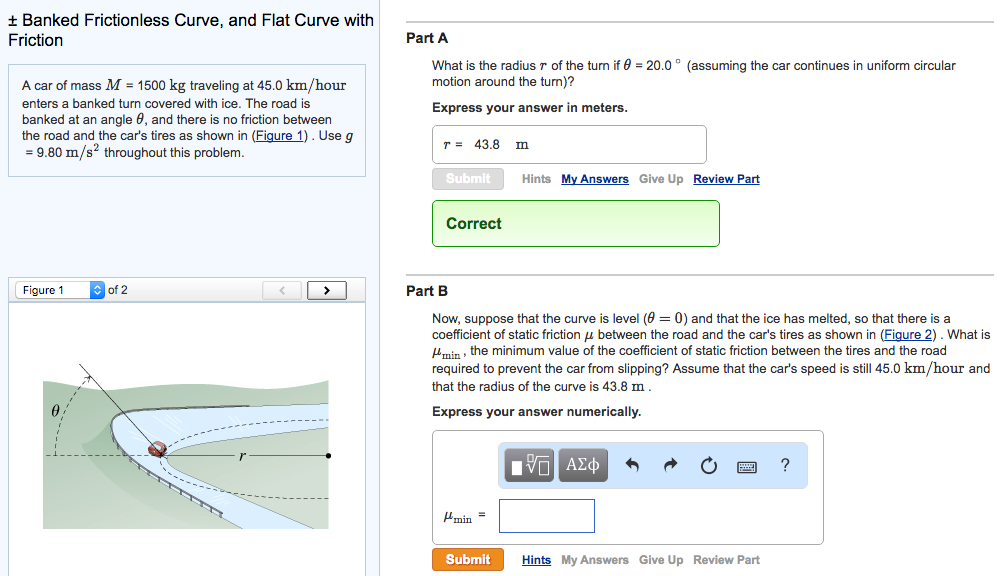PLEASE ANSWER PART B. THANKS! t Banked Frictionless Curve, and Flat Curve with Friction A car of mass M 1500 kg traveling at 45.0 km/hour enters a banked turn covered with ice. The road is banked at an angle 6, and there is no friction between the road and the car's tires as shown in (Figure 1). Use g 9.80 m/s2 throughout this problem. of 2 Figure 1 Part A What is the radius r of the turn if 0...

• ### Now, suppose that the curve is level (θ=0) and that the ice has melted, so that...

Now, suppose that the curve is level (θ=0) and that the ice has melted, so that there is a coefficient of static friction μ between the road and the car's tires as shown in (Figure 2) . What is μmin, the minimum value of the coefficient of static friction between the tires and the road required to prevent the car from slipping? Assume that the car's speed is still 50.0 km/hour and that the radius of the curve is 54.1...

• ### Now, suppose that the curve is level (θ=0) and that the ice has melted, so that...

Now, suppose that the curve is level (θ=0) and that the ice has melted, so that there is a coefficient of static friction μ between the road and the car's tires as shown in (Figure 2). What is μmin, the minimum value of the coefficient of static friction between the tires and the road required to prevent the car from slipping? Assume that the car's speed is still 45.0 km/hour and that the radius of the curve is 43.8 m.

• ### What is the radius of the turn of the angle is 20 degrees. ( assuming the...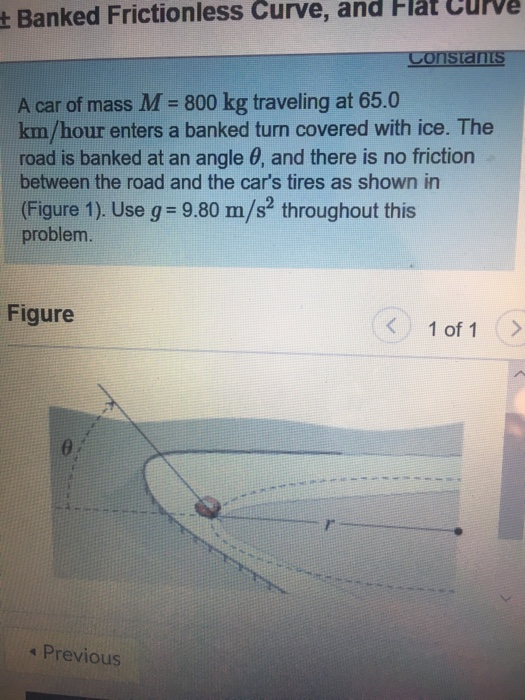What is the radius of the turn of the angle is 20 degrees. ( assuming the car continues in uniform circular motion around the turn? t Banked Frictionless Curve, and Hlat Curve A car of mass M- 800 kg traveling at 65.0 km/hour enters a banked turn covered with ice. The road is banked at an angle θ, and there is no friction between the road and the car's tires as shown in (Figure 1). Use g 9.80 m/s throughout...

• ### Banked Frictionless Curve, and Flat Curve with Friction Reviev A car of mass M - 1300...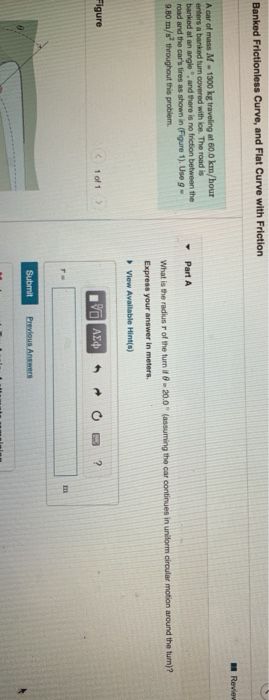Banked Frictionless Curve, and Flat Curve with Friction Reviev A car of mass M - 1300 kg traveling at 60.0 km/hour enfers a banked turn covered with ice. The road is banked at an angle and there is no friction between the road and the car's tires as shown in Figure 1). Use 9 - 9.B0 m/s throughout this problem. Part A What is the radius of the turn it 8 - 20.0 (assuming the car continues in uniform circular...